MS 095 PreAlgebra Test Two Name: ____________________

1. _______ Write the mixed number as an improper fraction: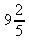2. _______ Write the improper fraction as mixed number: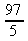3. _______ Reduce to lowest terms (simplify):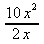4. _______ Multiply and simplify: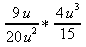5. _______ Calculate: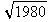6. _______ Simplify: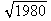7. _______ Find the Greatest Common Factor of 84 and 90.
8. _______ Find the Least Common Multiple of 84 and 90.
9. _______ Add and, if possible, simplify: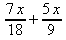10. _______ Solve for x: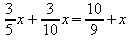11. _______ Round to the nearest hundredths: 271.8281828459045201817900760943
12. _______ Round to nearest hundreds: 271.8281828459045201817900760943
13. _______ Write in scientific notation: 300,000,000
14. _______ Write the scientific notation in standard form: 3.45 × 105
15. _______ Find h in the diagram using the Pythagorean Theorem: a² + b² = c²

16.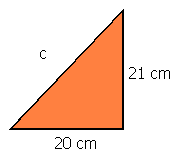_______ Find the volume of sphere with a diameter of 100 cm. Use p on your calculator for pi.
17. _______ Write as a repeating decimal in correct notation: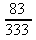18. _______ Does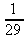repeat?
19. _______ Write as a repeating decimal in correct notation: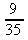20. _______ What is the result of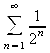?
21. _______ What is the result of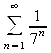?
22. What are the next three numbers in the sequence:
1, 1, 2, 3, 5, 8, 13, 21, _____, _____, _____
23. What are the next three numbers in the sequence:
1, 8, 27, 64, 125, 216, _____, ______, _____

1. _______________________ Find the prime factorization of 105710.
2. _______ The function for a falling sphere is: height = 4.16 * (time)² If a golf ball falls for 1.4 seconds, from what height was the golf ball dropped?
3. _______ Using the above function, determine the time for a sphere dropped 16.64 meters.
4. _______ Use the following diagram to determine the slope of the line shown. Use the x – y coordinate circled at the upper right.

5.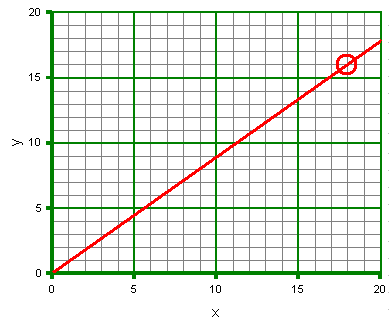_______ The function for the bounce height of a golf ball is:
bounce height = 0.63 * drop height. Find the bounce height for a golf ball dropped down the waterfall that has a height of 25.79 m.
6. _______ If a golf ball is found to have a bounce height of 100 centimeters, what was the drop height?
7. _______ The function for velocity is velocity = distance divided by time. Yesterday evening Kiumy won the 100 meter sprint with a time of 13.24 seconds. What was her velocity?
8. _______ The function for converting meters per second to miles per hour is:
miles per hour = 2.237 × meters per second
Find her speed in miles per hour.